# HSSlive: Plus One & Plus Two Notes & Solutions for Kerala State Board

## BSEB Class 12 Chemistry Solutions Textbook Solutions PDF: Download Bihar Board STD 12th Chemistry Solutions Book AnswersBSEB Class 12 Chemistry Solutions Textbook Solutions PDF: Download Bihar Board STD 12th Chemistry Solutions Book Answers

BSEB Class 12th Chemistry Solutions Textbooks Solutions and answers for students are now available in pdf format. Bihar Board Class 12th Chemistry Solutions Book answers and solutions are one of the most important study materials for any student. The Bihar Board Class 12th Chemistry Solutions books are published by the Bihar Board Publishers. These Bihar Board Class 12th Chemistry Solutions textbooks are prepared by a group of expert faculty members. Students can download these BSEB STD 12th Chemistry Solutions book solutions pdf online from this page.

## Bihar Board Class 12th Chemistry Solutions Books Solutions

 Board BSEB Materials Textbook Solutions/Guide Format DOC/PDF Class 12th Subject Chemistry Solutions Chapters All Provider Hsslive

2. Click on the Bihar Board Class 12th Chemistry Solutions Answers.
3. Look for your Bihar Board STD 12th Chemistry Solutions Textbooks PDF.
4. Now download or read the Bihar Board Class 12th Chemistry Solutions Textbook Solutions for PDF Free.

Find below the list of all BSEB Class 12th Chemistry Solutions Textbook Solutions for PDF’s for you to download and prepare for the upcoming exams:

### Bihar Board Class 12 Chemistry Solutions Intext Questions and Answers

Question 1.
Calculate the mass percentage of benzene (C6H6) and carbon tetrachloride (CCl4) if 22 g of benzene is dissolved in 122 g of CCl4.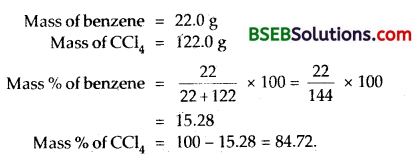Question 2.
Calculate the mole fraction of benzene in solution . containing 30% by mass in carbon tetrachloride.
Let the mass of the solution = 100.0 g
Mass of benzene = 30.0 g
Mass of Carbon tetrachloride (CCl4) = 70.0
Molar mass of benzene (C6H6) = 78 g mol-1
Molar mass of carbon tetrachloride (CCl4) = 154 g mol-1 ;Question 3.
Calculate the molarity of each of the following solutions (a) 30 g of CO (NO3)2. 6H2O in 4.3 L of solution (b) 30 mL of 0.5 M H2SO4 diluted to 500 mL.
(a) Volume of the solution = 4.3 L
Mass of Co (NO3)2.6H2O = 30.0 g
Molar mass of Co (NO3)2.6H2O = 59 + 2 x 14 + 6 x 16 + 6 x 18 = 291
Molality = 𝑆𝑡𝐿−1 M.Mass  = 304.3×291 = 0.024 M

(b) M1V1 = M2V2
30 mL of 0.5 M = M2 x 500
M2 = 30×5500 = 0.03 M

Question 4.
Calculate the mass of urea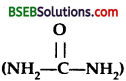required in making 2.5 kg of 0.25 molal aqueous solution.
Molar mass of urea= 60.0
1000 gm of aq. solution contains = 0.25 x 60 = 15.0 g
Mass of urea required to make 2500 g of solution
= 151000 x 2500 = 37.5 g

Question 5.
Calculate (a) molality, (b) molarity and (c) mole fraction of KI if the density of 20% (mass/mass) aqueous KI is 1.202 g mL-1.
(a) Molality (m)
M. Mass of KI = 39 + 127 = 166
80.0 g of water hold 20.0 g pf KI
1000.0 g of water holds 2080 x 1000 g of KI = 250 g of KI
molality =  St. per kg  M.Mass
= 2501661
= 1.5 cm

(b) Molarity(M)(c) Mole fraction of KIQuestion 6.
H2S, a toxic gas with rotten egg like smell, is used for the qualitative analysis. If the solubility of H2S in water at STP is 0.195 m, calculate Henry’s Law Constant.
Solubility of H2S in water = 0.195 m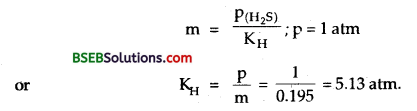Question 7.
Henry’s law constant for CO2 in water is 1.67 x 108 Pa at 298 K. Calculate the quantity of CO2 in 500 ml of soda water when packed under 2.5 atm CO2 pressure at 298 K.Question 8.
The vapour pressure of pure liquids A and B are 450 and 700 mm Hg at 350 K respectively. Find out the composition of the liquid mixture if total pressure is 600 mm Hg. Also find the composition of the vapour phase.
(i) Let the mole fraction of A in mixture = xA
∴ Mole fraction of B in mixture = 1 – xA
Ptotal = 600 mm Hg
A = 450mm Hg
B = 700 mm Hg
PTotal = P°A × xA + P°B(1 – xA)
= 450 xA + 700 (1 – xA )
600 = 450 xA + 700 – 700 xA
– 100 = – 250 xA
xA = Mole fraction of A in liquid mixture = 0.4
xA = Mole fraction of B in liquid mixture = 0.6

(ii) Composition in vapour phase
PA = PTotal × xA
where x’A is the mole fr. of A in V. phase
∴ PA = PAPTotal  = 0.4×450600 = 0.3
Mole fraction of A in Vapour phase = 0.3
Mole fraction of B in vapour phase = 0.7.

Question 9.
Vapour pressure of pure water at 298 K is 23.8 mm Hg. 50.0 g of urea (NH2 CONH2) is dissolved in 850 g of water. Calculate the vapour pressure of water for this solution and its relative lowering?
P° = 23.8 mm Hg, Ps = ?On solving ps = 23.38 mm Hg
∴ V.P. of water in the solution = 23.38 mm Hg
Lowering of V.P. = 23.80 – 23.38 = 0.42 mm Hg.

Question 10.
Boiling point of water at 750 mm Hg is 99.63°C. How much sucrose is to be added to 500 g of water so that it boils at 100°C.
kb for water = 0.52
w = ?, W = 500 g; ∆Tb = Ts – T0 = 100 – 99.63 = 0.37°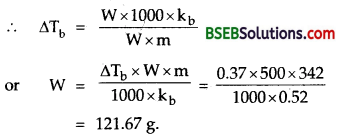Question 11.
Calculate the mass of ascorbic acid (Vitamin C, C6H8O6) to be dissolved in 75 g of acetic acid to lower its melting point by 1.5°C. Kf = 3.9 K kg mol-1.
∆Tf = 1.5°
w = 75 g
Let the mass of ascorbic acid = W
Molar mass of ascorbic acid = 176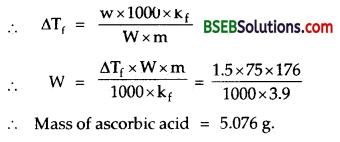Question 12.
Calculate the osmotic pressure in pascals exerted by a solution prepared by dissolving 1.0 g of polymer of molar mass 185,000 in 450 mL of water at 37°C.### Bihar Board Class 12 Chemistry Solutions Text Book Questions and Answers

Question 1.
Define the term solution. How many types of solutions are formed? Write briefly about each type with an example.
Solution – It is a homogeneous mixture of two or more components whose composition maybe varied within limits. There are nine types of solutions.

Types of Solutions: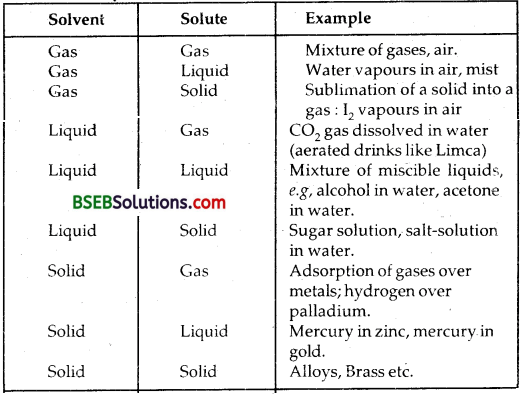Question 2.
Suppose a solid solution is formed between two substances, one whose particles are very large and the other whose particles are very small. What kind of solid solution is this likely to be?
The solid substance whose particles size is small enter into the cavities or interstices of the solid substance whose particles size is large to form an interstitial solid solution. Tungstun (W) and Carbon (C) form interstitial solid WC. Small solute particles fit into voids/ interstices in the host lattice. Tungstun carbide (WC) is extremely hard and is an example of such solid solution.

Question 3.
Define the following terms:
(i) Mole fraction
(ii) Molality
(iii) Molarity
(iv) Mass Percentage.
(i) Mole fraction – The ratio of the number of moles of a component to the total no. of moles in the solution is called its mole fraction.
Suppose there are two components A and B.
Let nA be the no. of Moles of A and nB be no. of moles of B.
xA Mole fraction of A = 𝑛𝐴𝑛𝐴+𝑛𝐵
xB Mole fraction of B = 𝑛𝐵𝑛𝐴+𝑛𝐵
So that xA + xA = 1
or xA = 1 – xB

(ii) Molality (m) – It is defined as the no. of moles of the solute dissolved in 1000 gm of the solvent.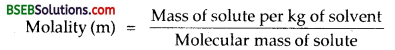(iii) Molarity (M) – It is defined as the number of moles of the solute dissolved per litre of the solution.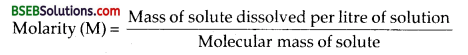(iv) Mass Percentage – The mass percentage of a component in a given solution is the mass of the component per 100 gm of the solution. If WA is the mass of component A and WB is the mass of the component B in a solution.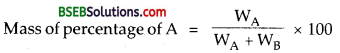Question 4.
Concentrated nitric acid used in the laboratory work is 68% nitric acid by mass in aqueous solution. What should be molarity of such sample of the acid if the density of the solution is 1.504 gmL-1?
Cone. HNO3 used in the laboratory = 68% by mass
i.e. 100 g of solution of HNO3 contains 68 g of HNO3
Density of the sample = 1.504 g ml-1
∴ Volume of 100 g of solution =  Mass  density  = 1001,504 = 66.489 mL
Molecular mass of HNO3 = 1 + 14 + 3 x 16 = 63
66.489 mL of solution contains HNO3 = 68.0 g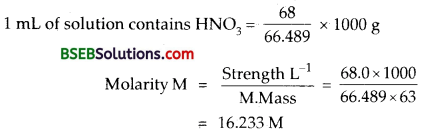Question 5.
A solution of glucose in water is labelled as i0% w/w, what would be the molality and mole fraction of each component in the solution? If the density of solution is 1.2 g mL-1, then what shall be the molarity of the solution?
Solution of glucose in water = 10% W/W
That means 100 g of solution of glucose in water contains 10 g of glucose in it.
1000 g of solution = 100 g of glucose
∴ Wt. of water = 1000 – 100 = 900 g
Now 900 g of water contain 100 g of glucose
1000 g of water contam = 100900 x 1000 = 10009 g
Strength of glucose (C6H12O6) = 18.0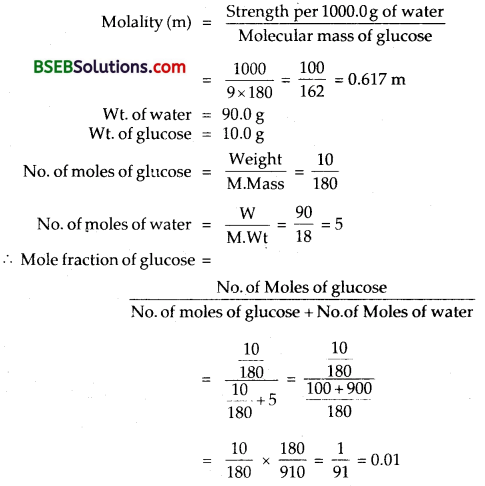Mole fraction of water = 1 – 0.01 = 0.99
To get molarity;
Vol. of 100 gm of solution = 𝑀𝑑 = 1001.2 = 83.333
[density of the solution = 1.2 g mL-1]
83.333 ml of solution contains 10 gm of glucose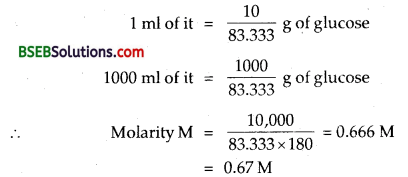Question 6.
How many mL of 0.1 M HCl are required to react completely with 1 g mixture of Na2CO3 and NaHCO3 containing equimolar amounts of both?
The wt. of the mixture of Na2CO, and NaHCO3 = 1.0 gm
Let the wt. of Na2CO3 in the mixture be x gm
∴ Wt. of NaHCO3 mixture = (1 – x) gm
No. of Moles of Na2CO3 in x gm of it = 𝑥106
[M. wt. of Na2CO3 = 106]
No. of Moles of NaHCO3 in (1 -x) gmof it
= 1−𝑥84
[M. wt. of NaHCO3 = 84]
Since the mixture is EQUIMOLAR
𝑥106 = 1−𝑥84
∴Wt. of Na2CO3 in the mixture = 106190
Wt. of NaHCO3 in the mixture = 1 – 106190 = 84190106 gm of Na2CO3 require 2 Mole of HC1 for complete neutralization
106190 gm of Na2CO3 will require = 2106 x 106190 = 2190 moles of HCL
84 gm of NaHCO3 requires for complete neutralization = 1 Mole of HCl
84190 gm of NaHCO3 requires = 184 x 84190 = 1190 Moles of HCl
Total No.of Moles required = 2190 x 1190 = 3190 Moles of HCl
∴ 100 ml of 3190 Molar HCl = ? ml of 0.1 HCl
∴ Vol.of 0.1 M HCl required = 1000 x 3190 x 10
= 157.8 mL

Question 7.
A solution is obtained by mixing 300 g of 25% solution and 400 g of 40% solution by mass. Calculate the mass percentage of the resulting solution.
Total mass of the solution = 700 g
300 g of 25% solution = 75 g of solute
400 g of 40% solution = 160 g of solute
Mass percentage of the resulting solution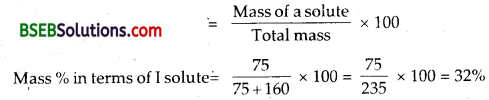Mass% in terms of II solute = 100 – 32 = 68%

Question 8.
An antifreeze solution is prepared from 222.6 g of ethylene glycol (C2H6O2) and 200 g of water. Calculate the molality of the solution. If the density of the solution is 1.072 g mL-1, then what shall be the molarity of the solution?
Mass of ethylene glycol (C2H6O2) in antifreeze solution = 222.6 g
Molecular mass of ethylene glycol = 2 x 12 + 6 x 1 + 2 x 16 = 62.0
Mass of water in the solution = 200 g
Now 200 g of water contains = 222.6 g of ethylene glycolQuestion 9.
A sample of drinking water was found to be severely contaminated with’chloroform. (CHCl3), supposed to be a carcinogen. The level of contamination was 15 ppm (by mass): (i) express this in percent by mass, (ii) determine the molality of chloroform in the water sample.
The level of contamination of water with CHCl3 (chloroform) = 15 ppm (by mass)
Now 106 parts by mass of water sample contains 15 parts of CHCl3
102 parts by mass of water sample contains 15106 x 102 parts of CHCl3
Thus contamination in water sample = 15 x 10-4 parts CHCl3

(ii) Molality of CHCl3 in contaminated water
1000 g of this sample = 1.5 x 10-2 g
Wt. of pure water = (1000 – 0.015)g = 999.985 g
∴ Molality (m) of CHCl3 in contaminated water = 0.015×1000999.985×18
= 8.33 x 10-4 m.

Question 10.
What role does the molecular interaction play in a solution of alcohol and water?
The combination of ethyl alcohol (C2H5OH) in water results in the formation of non-ideal solution showing positve deviation from Raoult’s Law. The interactions in the solution of ethyl alcohol in water are such that solute (ethyl alcohol) – solvent (water) interactions are weaker than solute-solute or solvent-solvent interactions in the two liquids before mixing. Escaping tendency in the solution becomes more than in pure liquids. Thus the vapour pressure of each component becomes more than expected from Raoult’s law.
In ethyl alcohol, molecules are held together due to hydrogen bonding as shown below :—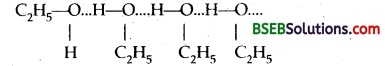When water is added to ethyl alcohol, water molecules tend to occupy the spaces betweeh ethyl alcohol molecules. Consequently, some hydrogen bonds in alcohols break and the attractive forces in alcohol molecules are weakened. In such solutions enthalpy on mixing increases. Therefore the process is ENDOTHERMIC. In such cases, there is an increase in volume also. Thus ∆HMix 0 and ∆VMix 0.
Thus heating will increase the solubility of such a solution.

Question 11.
Why do gases always tend to be less soluble in liquids as the temperature is raised?
The solubility of a gas decreases with the increase in temp, as some gas is expelled from its solution on heating or increasing the temperature.
In dissolving a gas ∆H is – ve, i.e., process is exothermic.
Gas + Solvent ⇌ Solution + heat
Applying Le-Chatelier’s principle, increase of temp will shift the equation, backwards, i.e., solubility of the gas decreases. There are exceptions also. Solubility of sparingly soluble gases like H2 and inert gases increases slightly with increase in temperature, especially in non- aqueous solvents such as acetone.

Question 12.
State Henry’s law and mention some important applications.
Henry’s Law : Effect of pressure on solubility of gases in liquids at a particular temperature is given by HENRY’S LAW. “The mass of a gas dissolved in a given volume of the liquid at a constant temperature is directly proportional to the pressure of the gas present in equilibrium with the liquid.”
Mathematically m ∝ p
m = kP
where m = mass of the gas dissolved in a unit volume of the solven
P = Pressure of the gas in equilibrium with the solvent
k = Constant called Henry’s Law constant.

Some Important Applications of Henry’s Law:

1. To increase the solubility of CO2 in soft drinks and soda-water, the bottle is sealed under high pressure.
2. Deep sea divers use oxygen diluted with less soluble helium as breathing gas to minimise the painful effects accompanying the decompression.
3. In lungs where oxygen is present in air with high partial pressure, haemoglobin combines with oxgyen to form oxohaemoglobin. In tissues where partial pressure of oxygen is low it releases oxygen for utilization in cellulsr activities.

Question 13.
The partial pressure of ethane over a solution containing 6.56 x 10-3 g of ethane is 1 bar. If the solution contains 5.00 x 10-2 g ethane, then what shall be the partial pressure of the gas?
Partial pressure of ethane (C2H6) = KH x Mole fraction of C2H6
where KH = Henry’s law constant
Let M be the no. of moles of the solvent.
∴ According to question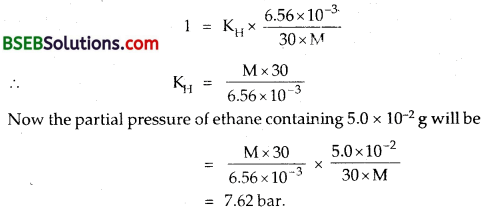Question 14.
What is meant by positive and negative deviations from Raoult’s law and how is the sign of ∆mixH related to positive and negative deviations from Raoult’s law?
Positive and negative deviations from Raoult’s law
Positive deviations – Here the partial vapour pressure of each component (say A and B) of a binary solution is greater than the vapour pressure as expected from Raoult’s law. Here the magnitude of the A-B interactions is less in the solution than the magnitude of interaction between A-A or B-B before mixing. In such solutions total vapour pressure of the solution is greater than expected from Raoult’s law.
In such solutions
PA > P°A × xA
PB > P°A × xB
Where PA, PB are partial V. PS of A and B in the solution P°A , P°B are their V.Ps in the pure state.
xA, xB are their mole fractions in the solution.
Here ∆HSolution > 0 or is positive. Such solutions are

• ethanol and n-hexane
• CCl4 and CHCl3

Solutions With Negative deviations – Here the partial vapour pressure of each component of solution is less than the vapour pressure as expected from Raoult’s Law. Here the magnitude of A-B interactions is more in the solution than that of A-A or B-B before solution formation. Thus escaping tendency decreases which results in the decrease of their partial vapour pressures. In such solutions, the total vapour pressure of the solution is also less than the vapour pressure as expected from Raoult’s Law.

An example of a solution with negative deviations is a solution of acetone and chloroform. In such components there is no hydrogen bonding when they are separate. However on mixing, hydrogen bonds are formed.For such non-ideal solution with negative deviations

1. PA < P°A × xA; PB < PB° xB
2. ∆HMixing = Negative
3. ∆VMixing = Negative

Other examples are:

1. Water + HCl
2. Water + HNOs3. CH3COOH and pyridine.

Question 15.
An aqueous solution of 2% non-volatile solute exerts a pressure of 1.004 bar at the normal boiling point of the solvent. What is the molar mass of the solute?
Mass of the solvent WA = 100 – 2 = 98g
Mass of the solvent WB = 2 g
Molecular mass of solvent MA = 18
Molecular mass of solute MB = ?
Change in Vapour Pressure = [P°A – PA = 1.013 – 1.004]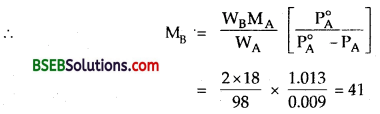Molar mass of the solute = 41 g mol-1

Question 16.
Heptane and octane form ideal solution. At 373 K, the vapour pressures of the two liquid components are 105.2 kPa and 46.8 kPa respectively. What will be the vapour pressure of a mixture of 26.0g of heptane and 35 g of octane?
Heptane and Octane form ideal solution
Mass of heptane (C7H16) = 26.0 g
Molecular mass of heptane = 7 x 12 + 16 x 1 = 100
No. of Moles of heptane = = 0.26
Mass of Octane (C8 H18) = 35 g
Molecular mass of Octane = 8 x 12 + 18 x 1 = 114
No. of moles of octane = 35114 = 0.30
Mole fraction of heptane = 2626+30 = 0.45
Mole fraction of octane = 0.55
Pheptane = 105.2 x 0.45 = 47.34
Poctane = 46.8 x 0.55 = 25.74
Vapour pressure of mixture = Pheptane + Poctane
= 47.54 + 25.74
= 73.08 kPa

Question 17.
The vapour pressure of water is 12.3 kPa at 300 K. Calculate vapour pressure of 1 molal solution of a non-volatile solute in it.
No. of Moles of the solute = 1
No. of Moles of whter in 1L of water = 100018 = 55.5 Moles
∴ Mole fraction of water = 55.51+55.5
(P°) Vapour pressure of water = 12.3 K Pa
∴ Vapour pressure of the solution
Ps = P° × x = 12.3 x 555565 K Pa
= 12.08 K Pa.

Question 18.
Calculate the mass of a non-volatile solute (molar mass 40 g mol-1) which should be dissolved in 114 g octane to reduce its vapour pressure to 80%.
Let the mass of the non-volatile solute = W
Its Molecular Mass = 40
Mass of octane = 114 gm
Molecular Mass of octane =114
Let the V.P. of pure octane = P°
Let the V.P. of solution octane = 80% of P° = 80100P°Simplifying m = 10.0
Mass of the solute = 10.0 g

Question 19.
A solution containing 30 g of non-volatile solute exactly in 90 g of water has a vapour pressure of 2.8 kPa at 298 K. Further, 18 g of water is then added to the solution, the new vapour pressure becomes. 2.9 kPa at 298 K. Calculate : (i) molar mass of the solute, (ii) vapour pressure of water of 298 K.
Let the vapour pressure of water at 298 K = P°
Let the molar mass of non-volatile solute = m
Molar mass of water (H2O) = 18
Mass of water = 90 g
Mass of solute = 30 g
Mole fraction of the non – volatile = 30/𝑚9018+30𝑚P°
= 3030+5𝑚
The vapour pressure of the solution = 2.8 k pa at 298 k
∴ 𝑃°–2.8𝑃° = 3030+5𝑚
18 g of water is added to the solution
∴ Mass of water = 90 + 18 = 108 g
Now
Mole fraction of the solute = 30/m10818+30 m = 3030+6𝑚
New relative lowering
𝑃°–2.9𝑃° = 3030+6𝑚 …(2)
Solving equations (1) and (2)
m = 34
∴ Molecular mass of the non-volatile solute = 34 g mol-1
Vapour pressure of water at 298 K (P°) = 3.4 kPa

Question 20.
A 5% solution (by mass) of cane sugar in water has freezing point of 271 K. Calculate the freezing point of 5% glucose in water if freezing point of pure water is 273.15 K.
Wt. of cane-sugar = 5.0 gm
(i) Wt. of Water (W) = 95.0
Molar Mass of cane-sugar (C12H22O11) = 342
Freezing Point of solute = Ts = 271 K
Freezing Point of water T0 = 273.15 K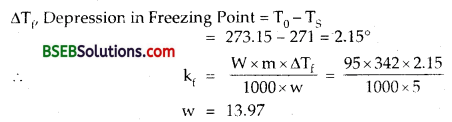(ii) Now wt. of glucose (w) = 5.0 g
Wt. of water (W) = 95.0 g
Molar mass of glucose m = 180∴ Freezing Point of the solution = 269.065 K.

Question 21.
Two elements A and B form compounds having formula AB2, and AB4. When dissolved in 20 g of benzene (C6H6), 1 g of AB lowers the freezing point by 2.3 K, whereas 1.0 g of AB4 lowers it by 1. K. The molar depression constant for benzene is 5.1 K kg mol-1. Calculate atomic masses of A and B.
Compounds formed by Elements A and B are AB2 and AB4
(i) Wt. of compound AB2 = lg
Wt. of benzene = 20.0 g
∆Tf = 2.3 k; kf = 5.1 k
Let the M. wt of AB2 = m1
m1 = 𝑤×1000×𝑘𝑖Δ𝑇𝑓×𝑊
= 1×1000×5.12.3×20
= 110.87

(ii) Let the M Mass compound AB4 = m2
Wt. of compound AB4 = 1
Wt . of benzene = 20
∆Tf = 1.3 k
m2 = 𝑤×𝑘𝑓×1000𝑊×Δ𝑇4
= 1×5.1×100020×1.3
= 196.15
m1 = At.wt.of A + 2 At.wt. of B
A + 2B = 110.87 … (1)
m2 = A + 4B = 196.15 … (2)
Subtracting (1) from (2)
2B = 196.15 – 110.87
B = 85.28/2
or A tomic Mass of B = 42.64
∴ Atomic Mass of A = 25.59

Question 22.
At 300 K, 36 g of glucose present per litre of its solution has an osmotic pressure of 4.98 bar. If the osmotic pressure of the solution is 1.52 bars at the same temperature, what would be its concentration?
Mass of glucose = 36 g L-1 of solution
Osmotic Pressure n = 4.98 bar = 4.91 atm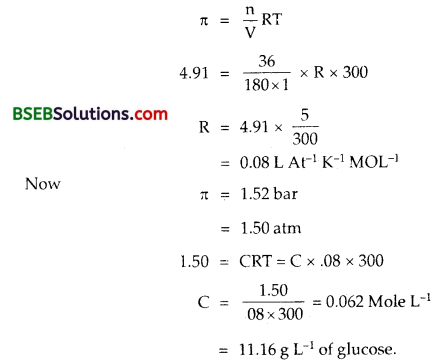Molar mass of glucose C6H12O6 = 180
∴ Concentration of glucose in Moles L-1 = 11.16180
= 0.063 M

Question 23.
Suggest the most important type of intermolecular attractive interaction in the following pairs, (i) n-hexane and n-octane, (ii) I2 and CCl4, (iii) NaClO4 and water, (iv) methanol and acetone, (v) acetonitrile (CH3CN) and acetone (C3HeO).
(i) n-hexane and n-octane form an ideal solution as solute- solvent interactions (attractive) are of the same magnitude as solute- soiute or solvent-solvent interactions before mixing.

(ii) I2 and CCl4 like dissolves like. Non-polar molecules of I2 are soluble in non-polar solvent like CCl4.

(iii) NaClO4 and water exihibit, negative dev lations from Raoult’s ia w, i.e., the forces of interactions between solute and solvent molecules are more than the solute-solute and solvent-solvent forces of interactions.

(iv) Methanol and acetone show positive deviations from Raoult’s [aiv. Here the forces of interaction between the two are less than those present in individual liquids.

(v) Acetonitrile and acetone also show positive deviations from Raoult’s law, i.e., solute-solvent forces of interaction are lower than those of solute-solute and solvent-solvent interactions. There are non-polar, non-polar interactions between them.

Question 24.
Based on solute-solvent interactions, arrange the following in order of increasing solubility in n-octane and explain. Cyclohexane, KCl, CH3OH, CH3CN.
n-Octane is a non-polar solvent. It will dissolve non-polar solute like cyclohexane to the maximum extent (like dissolves like) CH3CN is less polar than CH3OH. Therefore, CH3N is more soluble tnan CH3OH KCl is an ionic solid (maximum polar) and hence is least soluble in a non-polar solvent like n-octane. Thus the sequence of iacreasing solubility is as follows :
KCl < CH3OH < CH3CN < Cyclohexane

Question 25.
Amongst the following compounds, identify which are insoluble, partially soluble and highly soluble in water? (i) phenol, (ii) toluene, (iii) formic acid, (iv) ethylene glycol, (v) chloroform, (vi) pentanol.
Toluene and chloroform are insoluble (being non-polar) in a polar solvent like water. Phenol and pentanol are partially soluble in water because both form hydrogen bonding with water molecules.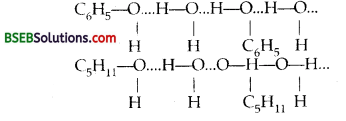Formic acid and ethyiene glycol are highly soluble in water because of their polar nature and excessive hydrogen bonding with water molecules. ,

Question 26.
If the density of some lake water is 1.25 g m L-1 and contains 92 g of Na+ ions per kg of water, calculate the molality of Na+ ions in the lake.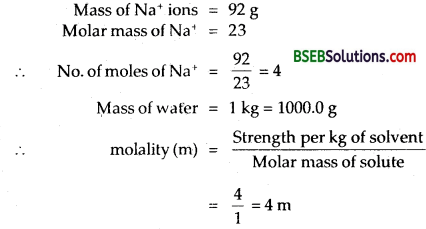Question 27.
If the solubility product of CuS is 6 x 10-16, calculate the maximum molarity of CuS in aqueous solution.
Solubility product (KSp) of CuS = 6 x 10-16
Let the solubility of CuS = S moles L-1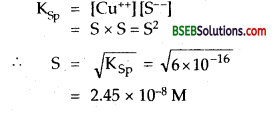∴ Maximum molarity (in a saturated solution) of CuS in aqueous solution = 2.45 x 10-8 M.

Question 28.
Calculate the mass percentage of aspirin (C9H8O4) in acetonitrile (CH3CN) when 6.5 g of C9H8O4 is dissolved in 450 g of CH3CN.
Mass of aspirin = 6.5 g
Mass of solvent, acetonitrile = 450.0 g
Total mass of aspirin and acetonitrile = 6.5 + 450.0 = 465.5 g
Mass percentage of aspirin =  Mass of aspirin  Total mass  x 100
= 6.5456.5 x 100 = 1.424.

Question 29.
Nalorphene (C19H21NO3), similar to morphine, is used to combat withdrawal symptoms in narcotic users. Dose of nalorphene generally given is 1.5 mg. Calculate the mass of 1.5 x 10-3m aqueous solution required for the above dose.
1.5 x 10-13 molal solution requires 1000.0 g of water
Mass of nalorphene dose = 1.5 mg = 1.5 x 10-13 g
Molar mass of C19H21NO3 = 311Question 30.
Calculate the amount of benzoic acid (C6H5COOH) required for preparing 250 mL of 0.15 M solution in methanol.
1000 mL of 1 M solution of benzoic acid contains 122 g of benzoic acid
1 mL of 1 M solution = 121000 of benzoic acid
250 mL of 1M solution = 121000 x 250 of benzoic acid
250 mL of 0.15 M solution = 121000 x 250 x 0.15
= 4.575 g of benzoic acid.

Question 31.
The depression in freezing point of water observed for the same amount of acetic acid, trichloroacetic acid and trifluoroacetic acid increases in the order given above. Explain briefly.
The lowering of vapour pressure of freezing point compared to that of the pure solvent. The freezing point of a Substance, the, solid phase is in dynamic equilibrium with the liquid phase.

The freezing point of a substance, may be defined as the temperature at which the vapour pressure of the substance in its liquid phase is equal to its vapour pressure of solid phase. A solution will freeze when its vapour pressure equals the vapour pressure of the pure solid solvent.

According to Raoult’s law, when a non-volatic solid is added to the solvent its vapour decreases and it would become equal to that of | solid solvent at lower temperature. The freezing point of the solvent decreases. Let T° be the freezing point of pure solvent Tf be its freezing point when non-volatile solute is dissolved in it.
∆Tf = T°f – Tf depression of freezing point.

Depression Constant – The unit of Kf is K kg. mol-1 values of Kf for some common solvent Acetic acid are listed in this way. If W2 gram of the solute having motor mass as present in gram of solvent produces the depression in freezing point ∆T1 of the solvent then molality,
The Solute is as
m = W2/M2 W1/1000
We can find out that the freezing point for the same amount of acetic acid, trichloroacetic acid and trifluoroacetic acid increase in same order.

Question 32.
Calculate the depression in the freezing point of water when 10 g of CH3CH2CHClCOOH is added to 250 g of water. Ka = 1.4 x 10-3, Kf = 1.86 K.kg mol-1.
Mass of CH3CH2CHClCOOH = 10 g = W (say)
Molar mass of CH3CH2CHClCOOH = 122.5 = m
Mass of water, W = 250 g
Molal Depression constant Kf = 1.86 K kg mol-1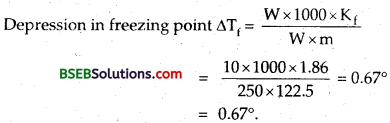Question 33.
19.5 g of CH2FCOOH is dissolved in 500 g of water. The depression in the freezing point of water observed is 1.0°C. Calculate the van’t Hoff factor and dissociation constant of fluoroacetic acid.
Mass of CH2FCOOH = 19.5 g
Molar mass of CH2FCOOH = 78.0
Mass of solvent water = 500.0 g
Kf for water = 1.86 K kg mol-1
∆Tf (observed) = 1.0°No.of Particles present at equilibrium = 1 – α + α + α = 1 + α
No.of particles taken = 1Question 34.
Vapour pressure of water at 293 K is 17.535 mm Hg. Calculate the vapour pressure of water at 293 K when 25 g of glucose is dissolved in 450 g of water.
Vapour pressure of water at 293 K = P° = 17.535 mm of Hg
Mass of glucose (C6H12O6) = 25 g
Molar mass of glucose = 180
Let Vapour Pressure of solution = Ps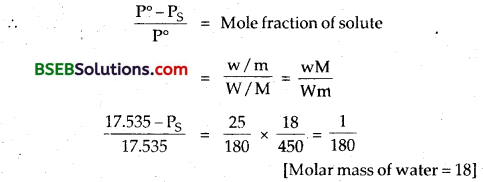Simplifying Ps = 3138.765/180 = 17.44
∴ Vapour pressure of solution = 17.44 mm of Hg

Question 35.
Henry’s law constant for the molality of methane in benzene at 298 K is 4.27 x 105 mm Hg. Calculate the solubility of methane in benzene at 298 K under 760 mm Hg.
According to Henry’s law
PA = KH × xA
pA = 760mmHg
KH = Henry’s law constant
= 4.27 x 105 mm.
760 = 4.27 x 105 × xA.
xA = 7604.27×105 x 10-5
∴ Mole fraction of solubility of methane in benzene at 760 mm Hg = 178 x 10-5.

Question 36.
100 g of liquid A (molar mass 140 g mol-1) was dissolved in 1000 g of liquid B (molar mass 180 g mol-1). The vapour pressure of pure liquid B was found to be 500 torr. Calculate the vapour pressure of pure liquid A and its vapour pressure in the solution if the total vapour pressure of the solution is 475 Torr.
Mass of liquid A = 100 g
Molar mass of liquid A = 140 g mol-1
Moles of A = 100140
Mass of liquid B = 1000 g
Molar mas qf liquid B = 180 g mol-1
Moles of B = 1000180Question 37.
Vapour pressure of pure acetone and chloroform at 328 K are 741.8 mm Hg and 632.8 mm Hg respectively. Assuming that they form ideal solution over the entire range of composition, plot ptotal Pchloroform, and Pacetone as a function of xacetone. The experimental data observed for different composition of mixture is: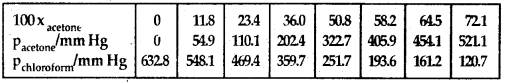Plot this data also on the same graph paper. Indicate whether it has positive deviation or negative deviation from the ideal solution.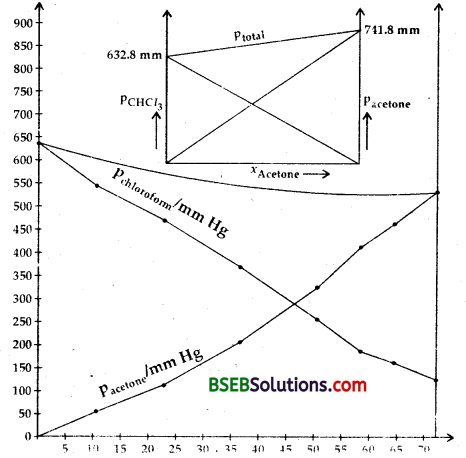Question 38.
Benzene and toluene form ideal solution over the entire range of composition. The vapour pressure of pure benzene and napthalene at 300 K are 50.71 mm Hg and 32.06 mm Hg respectively. Calculate the mole fraction of benzene in vapour phase if 80 g of benzene is mixed with 100 g of naphthalene.
No. of Moles of benzene = 8078 = 1.0256
No. of moles of naphthalene (C10H8) = 100128 = 0.78
Mole fraction of benzene = 1.02561.0256+0.78 = 0.5676
Mole fraction of napthalene = 0.4324
PT = P°A × xA + P°B × xB = 50.71 x 0.5676 + 32.06 x 0.4324
= 28.784 + 13.86 = 42.644 mm Hg
Now, from Dalton’s law of partial pressures
Partial vap. pr. of C6H6 = PTotal x Mole fr. of benzene in vapour phase
∴ 28.784 = 42.644 x Mole fr. of C6H6 in vapour phase
∴ Mole fraction of benzene in vapour phase = 28.78442.644 = 0.65

Question 39.
he air is a mixture of number of gases. The major components are oxygen and nitrogen with approximate proportion of 20% is to 79% by volume at 298 K. The water is in equilibrium with air at a pressure of 10 atm. At 298 K if the Henry’s law constants for oxygen and nitrogen at 298 K are 3.30 x 107 mm and 6.51 x 107 mm respectively, calculate the composition of these gases in water.
KH for O2 = 3.30 x 107 mm; KH for N2 = 6.51 x 107 mm Volume of O2 in air = 20% Vol. of N2 = 79% at 298 K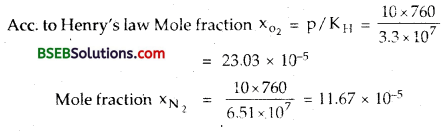Question 40.
Determine the amount of CaCl2 (i = 2.47) dissolved in 2.5 litre of water such that its osmotic pressure is 0.75 atm at 27°C.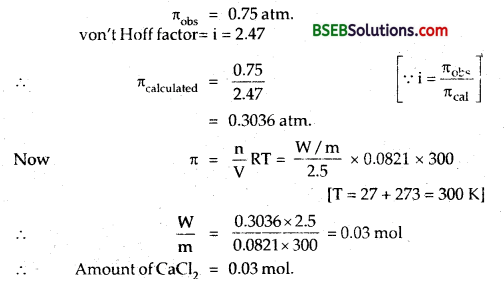Question 41.
Determine the osmotic pressure of a solution prepared by dissolving 25 mg of K2SO4 in 2 litre of water at 25°C, assuming that it is completely dissociated.
K2SO4 → 2K + + SO2-4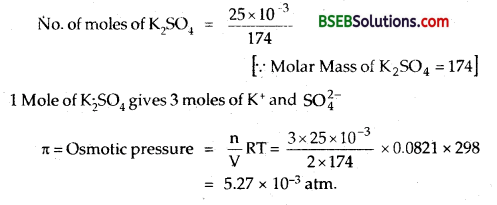### Bihar Board Class 12 Chemistry Solutions Additional Important Questions and Answers

Question 1.
What do you mean by 0,1 molar solution of a substance?
It means 0.1 mole of the substance is dissolved in 1 kg of the solvent.

Question 2.
What is the boiling point of an azeotrope of non-ideal solution showing positive deviations as compared to the boiling points of its components?
The B.Pt. of such an azeotrope is lower than that of its components.

Question 3.
What is the relationship between normality and molarity of a given solution of H2SO4?
Normality = 2 x Molarity

Question 4.
What is the effect of temperature of the molality of a solution? (H.S.B. 2005)
No. effect.

Question 5.
What is antifreeze? (P.S.B. 2005)
A substance like ethylene glycol which is added to water to lower its freezing point.

Question 6.
What is the sum of mole fractions of all the components in a three component system? (A.I.S.B. 2004)
The sum of the mole fraction of all the three components is = 1.

Question 7.
Oil and water do not mix. Why? (P.S.B. 1999)
Oil is non-polar, whereas water is polar.

Question 8.
What are constant boiling mixtures called?
Azeotropes.

Question 9.
What are isotonic solutions? Give one example.
Solutions having equal osmotic pressure, e.g., 0.1 M glucose and 0.1 M maltose solutions.

Question 10.
Why NaCl solution freezes at lower temperature than water but boils at higher temperature than water?
When a solute in dissolved in water, the vapour pressure decreases. Hence solution boils at a higher temperature, while solvent freezes at a lower temperature.

Question 11.
Give an example of a compound in which hydrogen bonding results in the formation of a dimer.
Benzoic acid (C6H5COOH) in benzene.

Question 12.
When is the value of van’t Hoff factor more than one?
It is > 1 when the solute undergoes dissociation in the solution.

Question 13.
Two liquids X and Y boil at 110°C and 130°C respectively. Which one of them has higher vapour pressure at 50°C?
Lower the B.Pt, more volatile the liquid. Hence X will have higher vapour pressure at 50°C.

Question 14.
Mention a large scale use of the phenomenon called ‘reverse osmosis’.
It is used in the desalination of water.

Question 15.
Give one example each of solid in gas and liquid in gas solution.
Iodine vapour in air, aerated drinks.

Question 16.
Define molality.
It is defined as the number of moles of a solute dissolved per 1000 g of a solvent.

Question 17.
Define molarity.
It is defined as the number of moles of a solute present in 1 litre of the solution.

Question 18.
What is van’t Hoff factor?
It is the ratio of the observed colligative property to the calculated colligative property.

Question 19.
What is van’t Hoff factor for KC1 in aqueous solution?
2.

Question 20.
Why is osmotic pressure considered to be a colligative property?
π = 𝑛𝑉RT; Osmotic pr. n depends upon n for the given volume of (he solution at a given temperature.

Question 21.
Why the vapour pressure of a solvent is lowered when a non-volatile solute is dissolved in it?
Some of the molecules of the solvent on the surface are replaced by the molecules of the solute which are non-volatile.

Question 22.
What type of liquids form ideal solutions?
Liquids having similar chemical structures and polarities.

Question 23.
Define Molal elevation constant/Ebullioscopic constant.
The elevation of B.pt which takes place when molality of the solution is unity.

Question 24.
State the law relating pressure ofa gas with its mole fraction in a solution.
Henry’s law.

Question 25.
Why does a solution of ethanol and cyclohexane show positive deviation from Raoult’s law? (P.S.B. 2005)
On adding cyclohexane, its molecules get in between the molecules of ethanol thus breaking the hydrogen bonds and reducing ethanol-ethanol interactions.

Question 1.
Explain why non-ideal solutions deviate from Raoult’s law?
Non-ideal solutions deviate from Raoult’s law because the new forces of interaction between the components (A – B interactions) are different from those present in the pure components (A-A or B-B interactions).

Question 2.
Under what conditions van’t Hoff factor is –

1. equal to one
2. greater than 1
3. less than 1.

1. i = 1, when the solute does not undergo any association or dissociation in the solution.
2. i > 1, when the solute undergoes dissociation in the solution.
3. i < 1, when the solute undergoes association in the solution.

Question 3.
Why an azeotropic mixture distils without any change in composition?
Such a mixture on boiling forms vapours in the same mole fraction of the two components as present in the liquid mixture.

Question 4.
Henry’s law constant (KH) for He, O2 and N2 at 273 K are 6.71 x 10-6 bar-1, 4.44 x 10-5 bar-1 and 1.87 x 10-5 bar-1 respectively. What will be the order of their solubility in water at the same pressure and why?
As KH are in bar-1, the Henry’s law will be in the form xA = KHPA. Thus, greater the value of KH, greater is the solubility. Thus solubility Will be in the order O2 > N2 > He.

Question 5.
Why dissolution of some solid compounds is exothermic while that of Some others is endothermic?
If solute-solvent interactions are stronger than solute-solute or solvent-solvent interactions, energy is released and the dissolution is exothermic. If solute-solvent interactions are weaker than solute-solute or solvent-solvent interactions, energy is absorbed and the dissolution is endothermic.

Question 6.
Why water cannot be separated completely from ethyl alcohol by fractional distillation?
Ehthyl alcohol and water (95.4% ethyl alcohol and 4.6% water) forms a constant boiling mixture or azeotrope boiling at 351.1 K. Hence both will distil together and thus further water cannot be removed by fractional distillation.

Question 7.
At a certain temperature, the vapour pressure of a mixture of benzene and toluene, expressed in mm, is represented by the equation P = 100 x + 60, where x is the mole fraction of benzene in the mixture. What will be the vapour pressure of the pure components at this temperature?
When x = 0; it is pure toluene and P = 60 mm.
When x = 1, these is only benzene; P = 100 x 1 + 60 = 160 mm.

Question 8.
What is the effect of temperature on molarity of a solution?
Molarity decreases, because volume of the solution increases with increase in temperature, but number of moles of the solute remains the same (assuming it to be a non-volatile).

Question 9.
Which aqueous solution has higher concentration: 1 Molar or 1 molal solution of the same electrolyte. Give reason.
1 M (molar) has higher concentration than 1 molal. This is because 1 molal sol = 1 mole in 1000 g of the solvent (water, ∵ d = 1 g cc-1). But 1 M sol = 1 mole in 1000 cc of the solution which contains less than 1000 cc of solvent, because solute is also present in it. Hence 1000 cc of the solvent will contain more than 1 mole of the solute.

Question 10.
Which of the 1 molar and 1 molal solution is more concentratred and why?
For aqueous solutions, the answer is same as in above. If the solution is non-aqueous 1 molar solution can be >< 1 depending upon the density of the solution.

Question 11.
When and why is molality preferred over molarity in handling solutions in chemistry? (DSB 1998, HPSB 2002)
Molality is preferred when studies are made independent of temperature. This is because molality involves masses which do not change with temperature.

Question 12.
10 cc of a liquid A were mixed with 10 cc of liquid B. The volume of the resulting solution was found to be 19.9 cc. What do you conclude? (A.I.S.B. 2005)
Decrease in volume means stronger intermolecular forces of attraction on mixing. This implies, that the solution shows negative deviation from Raoult’s law.

Question 13.
Two liquids A and B on mixing produce a warm solution. Which type of deviation from Raoult’s law does it show?
Warming up of the solution on mixing in solution indicates that the process is exothermic (∆Hmix is – ve). This implies that the solution shows negative deviation from Raoult’s law.

Question 14.
Equimolal solutions of NaCl and BaCl2 are prepared in water. Freezing point of NaCl is found to be – 2°C. What freezing point do you expect for BaCl2 solution?Question 15.
Which colligative property is preferred for the molar mass determination of macromolecules and why?
Osmotic pressure determination is better suited for molar mass determination of macromolecules like proteins, polymers etc. Changes observed in other colligative properties for such solutions are usually very low and can’t be measured easily.

Question 16.
Sodium chloride or calcium chloride is used to clear snow from the roads. Why? (H.P.S.B. 2002, H.S.B. 2005)
These salts depress the freezing point of water to such an extent that it cannot freeze to form ice. Hence, it melts off easily at the prevailing temperature.

Question 17.
Explain the significance of Henry’s constant (RH). At the same temperature, hydrogen is more soluble in water than helium. Which of them will have a higher value of KH and why?
Significance of KH As pA = KH xA. Thus, at constant temperature for the same partial pressure of different gases, xA ∝ 1 KH. In other words, solubility is inversely proportional to Henry’s constant of the gas. Higher the value of KH, lower is the solubility of the gas. As H2 is more soluble than He, H2 will have lower value of KH than that of He.

Question 18.
What are colligative properties? Mention then.
Colligative properties are those which depend upon the number of particles (atoms, molecules or atoms) of the solute and not on their nature. There are 4 colligative properties.

• Relative lowering of vapour pressure.
• Elevation in Boiling point.
• Depression in Freezing point.
• Osmosis or osmotic pressure.

Question 19.
What happens when blood cells are placed in pure water?
Due to osmosis, water molecules move into blood cells through the cell walls. As a result blood cells swell and may even burst.

Question 20.
What do you expect to happen when Red Blood Corpuscles (RBC’s) are placed in. (i) 1 % NaCl solution, (ii) 0.5% NaCl solution?

1. They will shrink due to plasmolysis.
2. They will swell and may even burst.

This is because RBC’s are isotonic with 0.91% NaCl Solution.

Question 1.
What are the methods available to measure concentration of solutions. Discuss them in brief.
1. Mass Percentage may be defined as the no. of parts by¬mass of solute per 100 parts by mass of solution.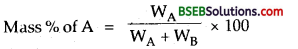2. Volume Percentage-Number of parts by volume of a solute per 100 parts by volume of solution.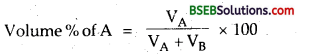3. Normality (N) – It is defined as the number of gm equivalents of a solute dissolved per litre of the solution.4. Molarity (M) – It is defined as the number of gm moles of the solute dissolved per litre of the solution.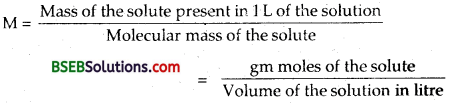5. Molality (m) – It is defined as the number of gm moles of the solute dissolved per 1000 gm of the solvent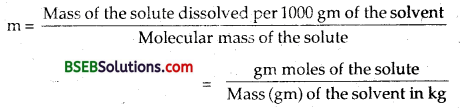6. Mole Fraction – It is defined as the ratio of the number of moles of a component to the total number of moles in the solution.
If, for a binary solution, nA and nB represent the number of moles of the solute and solvent, then,Knowing the mole fraction of one, that of the other can be determined7. Parts per million (ppm) – It is the number of parts by mass of the solute per million parts by mass of the solution.Question 2.
Why do we get abnormal molecular masses from colligative properties? What is Van’t Hoff factor? How will you explain the cases of association and dissociation of the solute in such cases?
The relation between colligative properties and molecular mass of the solute is based upon the following assumptions.

• The solution is dilute, so that Raoult’s Law is obeyed.
• The solute neither undergoes dissociation or association in solution.

When we measure the colligative properties of the solutions where above assumptions are not valid we. find discrepencies between the observed and calculated values of colligative properties.

These anomalies are primarily due to:

1. Association of solute molecules and
2. Dissociation of the solute particles.

1. Association of solute molecules – Certain solutes in solution are found to associate. This eventually leads to a decrease in the number of molecular particles in the solutions. Thus, it results in a decrease in the values of colligative properties. As already discussed, the colligative properties are inversely related to the molecular mass, colligative property ∞ 1 molecular mass of solute  therefore, higher values are obtained for molecular mass of solute
molecular masses than normal value for unassociated molecules.

For example, acetic acid dissolved in benzene shows a moleculaf mass of 12Q (normal molecular mass is 60). Similarly, benzoic acid dissolved in benzene is found to have molecular mass double of its normal molecular mass. This is explained by the fact, that both acetic acid and benzoic acid form dimers in solution due to hydrogen bonding.2. Dissociation of solute molecules – A number of electrolytes dissociate in solution to give two or more particles (ions). Therefore, the number of solute particles, in solutions of such substances, is more than the expected value. Accordingly, such solutions exhibit higher values of colligative properties. Since colligative properties are inversely proportional to molecular masses, therefore, molecular masses of such substances as calculated from colligative properties will be less than their normal values.

For example, KC1 dissociates into K+ and Cl ions when dissolved in water. So the number of solute particles in its solution would be double the number of particles if no dissociation had taken place. Hence, it is expected to have molecular mass, i.e., 74.52 or 37.25. However, the molecular mass of KCl is found to be 40.3 by studies of depression in freezing point.

The difference in the two values is due to the fact that there are strong attractive forces present in the oppositely charged ions of the strong electrolyte in solution. These electrical forces hold together a number of the ion pairs. Thus, such electrolytes are incompletely dissociated and the number of ions formed ini the solution is not exactly the double, but is somewhat less. Consequently there is difference in the value of the molecular masses.

Van’t Hoff’s Factor – In 1886, Van’t Hoff introduced a factor ‘i’ called Van’t Hoff’s factor, to express the extent of association or dissociation of solutes in solution. It is ratio of the normal and observed molecularm asses of the solute i.e.,In case of association, observed molecular mass being more than the normal, the factor i has a value less than 1. But in case of dissocation, the Van’t Hoff’s factor is more than 1 because the observed molecular mass has a lesser value than the normal molecular mass. In case there is no dissociation the value of ‘i’, becomes equal to one.

Since colligative properties are inversely proportional to molecular masses, the Van’t Hoff’s factor may also be written as: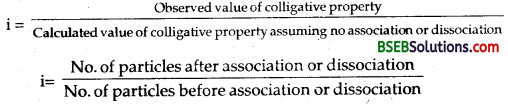Introduction of the Van’t Hoff factor modifies the equations for the colligative properties as follows:From the value of T, it is possible to calculate degree of dissociation or degree of association of substance in solution. Let us first consider the case of dissociation.

Degree of Dissociation – It is defined as the fraction of total molecules which dissociates into simpler molecules or ions.

In order to calculate the degree of dissociation let us suppose that each molecule of a substance on dissociatioh gives m particles and that a is the degree of dissociation. Then for each mole of the substance dissolved
Number of moles dissociated = α
Number of moles left undissociated = 1 – α
Total number of particles at equilibrium = (1 – α) + mα
Van’t Hoff’s factor =For the electrolyte of the type AB, such as NaCl, KC1 number of particles in solutions = 2
∴ α = i – 1
Similarly for the electrolyte of the type AB2 like CaCl2 number of particles in the solution = 3
CaCl2 ⇌ Ca++ + 2Cl
Hence α = i – 12
Similarly it is possible to calculate the degree of association (a) from the knowledge of i.

Degree of association – It is defined as the fraction of the total number of molecules which associate or combine together resulting in the formation of bigger molecules.
In order to calculate the degree of association, let us suppose that if m particles of the solute associate to form one big particle and a is the degree of association as given in the following equation.
m’A ⇌ [A°l
1 – α = α𝑚
Number of moles after association (1 – α) of A
Number of moles after association [A]m = α𝑚
N.D. Study Material Based On Total number of particles in equilibrium = (1 – α + α𝑚 ] moles
Thus the Van’t Hoff factor i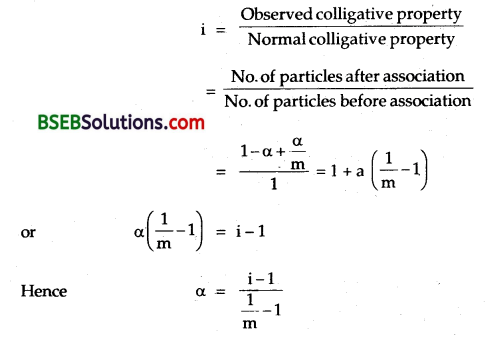## Bihar Board Class 12th Chemistry Solutions Textbooks for Exam Preparations

Bihar Board Class 12th Chemistry Solutions Textbook Solutions can be of great help in your Bihar Board Class 12th Chemistry Solutions exam preparation. The BSEB STD 12th Chemistry Solutions Textbooks study material, used with the English medium textbooks, can help you complete the entire Class 12th Chemistry Solutions Books State Board syllabus with maximum efficiency.

## FAQs Regarding Bihar Board Class 12th Chemistry Solutions Textbook Solutions

#### Can we get a Bihar Board Book PDF for all Classes?

Yes you can get Bihar Board Text Book PDF for all classes using the links provided in the above article.

## Important Terms

Bihar Board Class 12th Chemistry Solutions, BSEB Class 12th Chemistry Solutions Textbooks, Bihar Board Class 12th Chemistry Solutions, Bihar Board Class 12th Chemistry Solutions Textbook solutions, BSEB Class 12th Chemistry Solutions Textbooks Solutions, Bihar Board STD 12th Chemistry Solutions, BSEB STD 12th Chemistry Solutions Textbooks, Bihar Board STD 12th Chemistry Solutions, Bihar Board STD 12th Chemistry Solutions Textbook solutions, BSEB STD 12th Chemistry Solutions Textbooks Solutions,
Share: